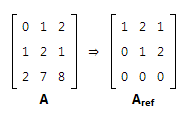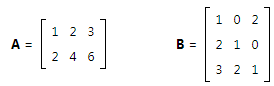Courses

# Rank of Matrices - Matrix Algebra, CSIR-NET Mathematical Sciences Mathematics Notes | EduRev

## Mathematics : Rank of Matrices - Matrix Algebra, CSIR-NET Mathematical Sciences Mathematics Notes | EduRev

The document Rank of Matrices - Matrix Algebra, CSIR-NET Mathematical Sciences Mathematics Notes | EduRev is a part of the Mathematics Course Mathematics for IIT JAM, CSIR NET, UGC NET.
All you need of Mathematics at this link: Mathematics

The Rank of a Matrix

You can think of an r x c matrix as a set of r row vectors, each having c elements; or you can think of it as a set of c column vectors, each having r elements.

The rank of a matrix is defined as (a) the maximum number of linearly independent column vectors in the matrix or (b) the maximum number of linearly independent row vectors in the matrix. Both definitions are equivalent.

For an r x c matrix,

• If r is less than c, then the maximum rank of the matrix is r.
• If r is greater than c, then the maximum rank of the matrix is c.

The rank of a matrix would be zero only if the matrix had no elements. If a matrix had even one element, its minimum rank would be one.

How to Find Matrix Rank

In this section, we describe a method for finding the rank of any matrix. This method assumes familiarity withechelon matrices and echelon transformations.

The maximum number of linearly independent vectors in a matrix is equal to the number of non-zero rows in its row echelon matrix. Therefore, to find the rank of a matrix, we simply transform the matrix to its row echelon form and count the number of non-zero rows.

Consider matrix A and its row echelon matrix, Aref. Previously, we showed how to find the row echelon form for matrix A.Full Rank Matrices

When all of the vectors in a matrix are linearly independent, the matrix is said to be full rank. Consider the matrices A and B below.Notice that row 2 of matrix A is a scalar multiple of row 1; that is, row 2 is equal to twice row 1. Therefore, rows 1 and 2 are linearly dependent. Matrix A has only one linearly independent row, so its rank is 1. Hence, matrix A is not full rank.

Now, look at matrix B. All of its rows are linearly independent, so the rank of matrix B is 3. Matrix B is full rank.

Offer running on EduRev: Apply code STAYHOME200 to get INR 200 off on our premium plan EduRev Infinity!

197 docs

,

,

,

,

,

,

,

,

,

,

,

,

,

,

,

,

,

,

,

,

,

,

,

,

;